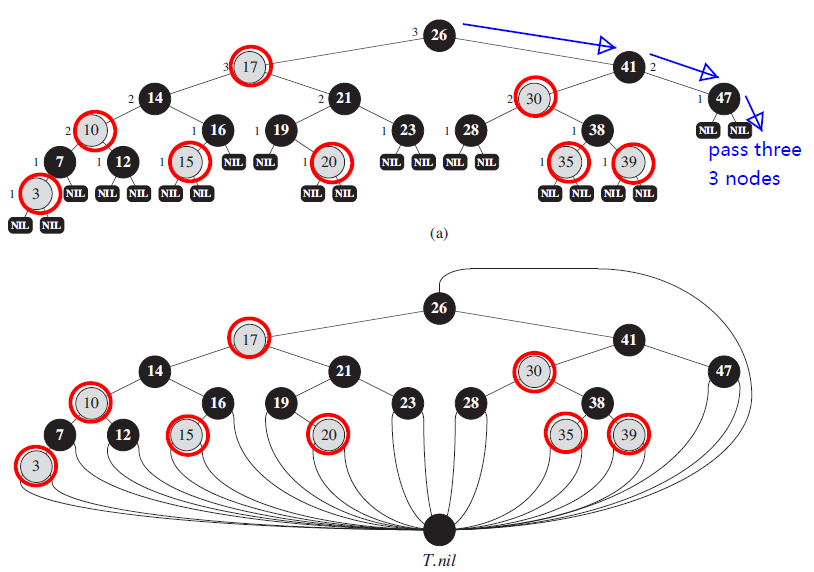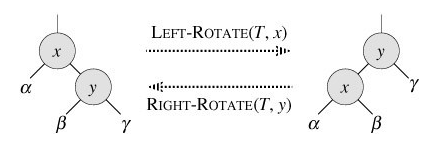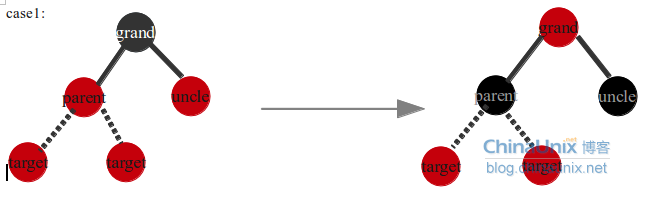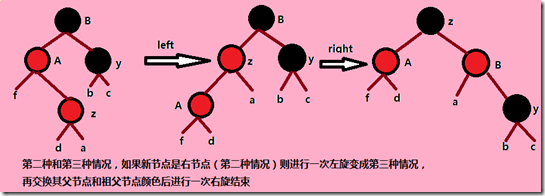# 算法导论学习---红黑树详解之插入(C语言实现)

#define RED 1
#define BLACK 0

///红黑树结点定义,与普通的二叉树搜索树相比多了一个颜色域
typedef struct node
{
int key,color; ///定义1为红,0为黑
node *p,*left,*right;
node(){
color=BLACK; ///默认结点颜色为黑
}
}*RBTree;

1).每个结点或是红色或是黑色。
2).根结点是黑色的。
3).每个叶结点是黑色的
4).如果一个结点是红色的，那么它的两个子节点都是黑色的。
5).对于每个结点，从该结点出发到其所有后代叶结点的简单路径上均包含相同数目的黑结点。///左旋:对具有任意具有右孩子的结点可以进行
///传入要选择的结点指针的引用
///旋转以后x的右孩子y取代了x,而x成为y的左孩子,y的左孩子成为x的右孩子
///下面的代码就是完成这三个部分
void LeftRotate(RBTree x)
{
RBTree y=x->right; ///y表示x的右孩子
x->right=y->left; ///第一步:将x的右孩子设为y的左孩子
if(y->left!=Nul)
y->left->p=x;

y->p=x->p;  ///更改y的父结点为x的父结点
if(x->p==Nul)    ///第二步:y替代x,需要分情况讨论
rt=y; ///x原来是根结点,则设y为根结点
else if(x==x->p->left)
x->p->left=y;   ///更改y为x父结点的左孩子
else
x->p->right=y; ///更改y为x父结点的右孩子

y->left=x; ///第三步:将x设为y的左孩子
x->p=y;
}

///右旋:对任何具有左孩子的结点可以进行
///传入要右旋的结点指针的引用
///旋转以后结点x被左孩子y替换,x成为y的右儿子,y的右孩子成为x的左孩子
void RightRotate(RBTree x)
{
RBTree y=x->left; ///y表示x的左孩子
x->left=y->right; ///第一步:x的左孩子更改为y的右孩子
if(y->right!=Nul)
y->right->p=x;

y->p=x->p;  ///更改y的父结点为x的父结点
if(x->p==Nul) ///第二步:y替代x,需要分情况讨论
rt=y;  ///x原来为根结点,指定y为新根结点
else if(x==x->p->left)
x->p->left=y;  ///更改x父结点的左孩子为y
else
x->p->right=y; ///更改x父结点的右孩子为y

y->right=x; ///第三步:更改y的右结点为x
x->p=y;
}

///红黑树的插入
///RB插入函数与普通的BST的插入函数只是稍微有点不同
///我们将原来的null换成了Nul结点,然后对新加入的结点,染成红色
///然后调用RBInsertFixUp函数进行调整,使得红黑树的性质不被破坏
void RBInsert(int key)
{
RBTree z=new node;
z->color=RED;
z->key=key;
z->p=z->left=z->right=Nul;
RBTree y=Nul;
RBTree x=rt;
while(x!=Nul) ///按照二叉搜索树的性质寻找z的插入点
{
y=x;
if(z->key<x->key)
x=x->left;
else
x=x->right;
}
z->p=y;
if(y==Nul)///插入的是根节点
rt=z;
else if(z->key<y->key)
y->left=z;
else
y->right=z;
RBInsertFixUp(z); ///插入红色结点可能违反了红黑树的某些性质,调用调整函数进行调整
}

1)情况1：y的颜色为红色2)：y的颜色为黑

(1).z是其父结点的左孩子

(2).z是其父结点的右孩子///红黑树插入调整函数
///我们将插入结点染成红色,可能违反了性质4,所以要进行调整
///调整的过程其实就是根据不同的情况进行分类讨论,不断转换的过程
///最后转成可以通过染色和旋转恢复性质的情况
void RBInsertFixUp(RBTree z)
{
///在下面的代码中z结点总是违反性质4的那个结点
while(z->p->color==RED) ///x是红色,它的父结点也是红色就说明性质4被违反,要持续调整
{
///下面的过程按x->p是其祖父结点的左孩子还是右儿子进行分类讨论
if(z->p==z->p->p->left) ///父结点是其祖父结点的左孩子
{
RBTree y=z->p->p->right;  ///表示z的叔结点

///下面按y的颜色进行分类讨论
if(y->color==RED)
{///如果y是红色并z的祖父结点一定是黑色的,这时我们通过下面的染色过程
///在保证黑高不变的情况下(性质5),将z在树中上移两层,z=z->p->p
z->p->color=BLACK;
y->color=BLACK;
z->p->p->color=RED;
z=z->p->p;///如果上移到根节点或某个父结点不为红的结点就可以结束循环了
}
else   ///叔结点为黑色
{ ///此时要根据z是其父结点的左孩子还是右孩子进行分类讨论
///如果z是左孩子则可以直接可以通过染色和右旋来恢复性质
///如果z是右孩子则可以先左旋来转成右孩子的情况

if(z==z->p->right)
{
z=z->p;
LeftRotate(z); ///直接左旋
}
///重新染色,再右旋就可以恢复性质
z->p->color=BLACK;
z->p->p->color=RED;
RightRotate(z->p->p);
}
}
else///父结点是祖父结点的右孩子
{
RBTree y=z->p->p->left;  ///叔结点
if(y->color==RED)
{
z->p->color=BLACK;
y->color=BLACK;
z->p->p->color=RED;
z=z->p->p;
}
else
{///右儿子的时候可以直接左旋,重新调色恢复性质
///左儿子可以先右旋成右儿子再处理
if(z==z->p->left)
{
z=z->p;
RightRotate(z);
}
z->p->color=BLACK;
z->p->p->color=RED;
LeftRotate(z->p->p);
}
}
}
///将根节点染成黑色，是必要的步骤；处理两种情况
///1.第一次插入根结点被染成红色的情况
///2.和在上面的循环中根节点可能被染成红色的情况
rt->color=BLACK;
}